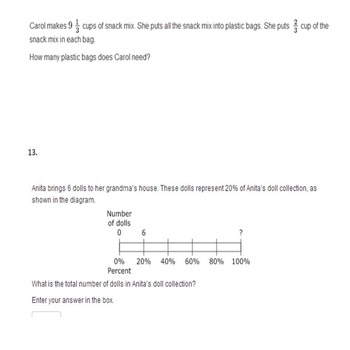# 6th Grade Math Test Year Bundle: Entire Year- 9 Units- All Common Core StandardsSubject
Resource Type
File Type
Zip (3 MB|103 pages)
Standards
\$20.00
List Price:
\$27.00
You Save:
\$7.00
• Product Description
• Standards
This math test bundle includes all the 9 units for the entire 6th grade year. It addresses all the common core standards and uses word problems, MCAS questions and PARCC questions within the tests.

These tests are sold individually for \$3.00 each but you can save \$7.00 by buying them all together. In the preview I have put my three most popular tests. Answer keys are provided for every test.

The units are as followed:
Decimals and Number Theory
Integers
Rational Numbers
Ratios and Rate
Percents and Division of Fractions
Expressions and Equations
Inequalities and Linear Equations
Geometry
Statistics

https://www.teacherspayteachers.com/Store/Going-From-Good-To-Great

**This is also included in a year-long math test bundle that includes 9 unit tests, study guides, test with goals and challenging test covering all the 6th grade Common Core Math Standards. Get the bundle here:
6th Grade Math Test, Study Guide, Test with Goals, Challenging Test Year Bundle
Use ratio reasoning to convert measurement units; manipulate and transform units appropriately when multiplying or dividing quantities.
Find a percent of a quantity as a rate per 100 (e.g., 30% of a quantity means 30/100 times the quantity); solve problems involving finding the whole, given a part and the percent.
Solve unit rate problems including those involving unit pricing and constant speed. For example, if it took 7 hours to mow 4 lawns, then at that rate, how many lawns could be mowed in 35 hours? At what rate were lawns being mowed?
Make tables of equivalent ratios relating quantities with whole-number measurements, find missing values in the tables, and plot the pairs of values on the coordinate plane. Use tables to compare ratios.
Use ratio and rate reasoning to solve real-world and mathematical problems, e.g., by reasoning about tables of equivalent ratios, tape diagrams, double number line diagrams, or equations.
Total Pages
103 pages